Courses

# Refraction of Light and Total Internal Refraction Class 10 Notes | EduRev

## Science Class 10

Created by: Dr Manju Sen

## Class 10 : Refraction of Light and Total Internal Refraction Class 10 Notes | EduRev

The document Refraction of Light and Total Internal Refraction Class 10 Notes | EduRev is a part of the Class 10 Course Science Class 10.
All you need of Class 10 at this link: Class 10

REFRACTION OF LIGHT
The bending of a ray of light as it passes from one medium to another is called refraction. It is due to change in velocity of light while travelling from one medium to another.
(i) The maximum velocity of light is 3 * 108 m/sec in vacuum or air.
(ii) The velocity is less in denser medium.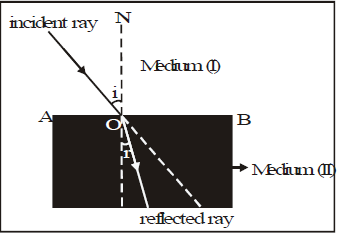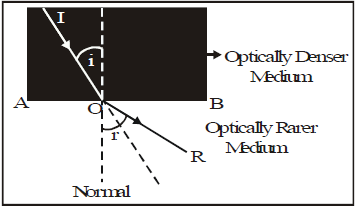THE LAWS OF REFRACTION
(i) The ratio of sine of the angle of incidence to the sine of the angle of refraction for a particular pair of media is constant. Thus if the angle of incidence is i, and that of refraction is r, then Sini/sinr = constant = μ it is known as Snell's law.
(ii) The incident ray, the refracted ray and the normal at the point of incidence point, all lie in the same plane.
Relative refractive index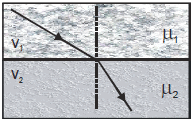When light passes from one medium to the other, the refractive index of medium 2 relative to 1 is written as 1μ and is defined as μ21 or 1μ2 or 1m 2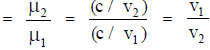Bending of light ray
According to Snell's law, μ sin i = μsin r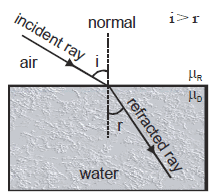(i) If light passes from rarer to denser medium:
μ = μ and μ= μ
so that,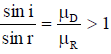⇒ ∠i > ∠r
In passing from rarer to denser medium, the ray bends towards the normal.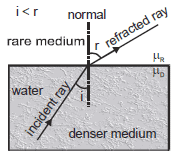(ii) If light passes from denser to rarer medium μ1= μand μ= μ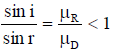⇒ ∠ i < ∠r
In passing from denser to rarer medium, the ray bends away from the normal.
Refractive index depends on nature and density of medium and colour of light refractive index is maximum for violet and minimum of red light.

APPARENT DEPTH AND NORMAL SHIFT
If a point object in denser medium is observed from rarer medium and boundary is plane, then from Snell's law we have μsin i = μsin r ......(i)
If the rays OA and OB are close enough to reach the eye.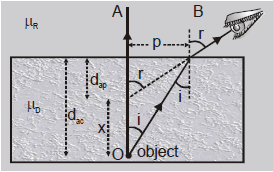sin i ~ tan i = p/dac and sin r ~ tan r = p/dap

So that eqn. (i) becomes dac = actual depth dap = apparent depth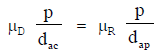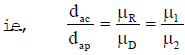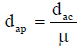so dap < dac ......(ii)
The distance between object and its image is called normal shift (x)

x = dac - dap  [Q ]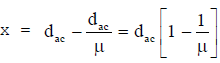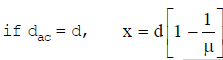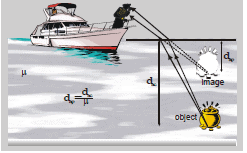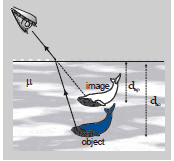Object in a rarer medium is seen from a denser medium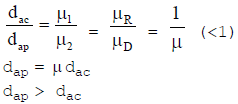A high flying object appears to be higher than in reality.

x = dap - dac  ⇒ x = [μ - 1] dac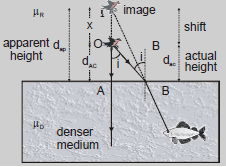Lateral Shift: The perpendicular distance between incident and emergent ray is known as lateral shift.
Lateral shift d = BC and t = thickness of slab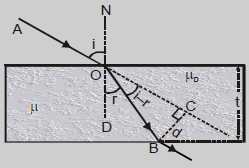In ΔBOC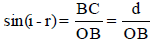⇒ d = OB sin(i – r)                ....(i)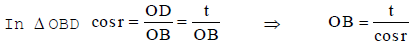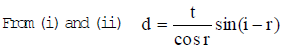Some Illustrations of Refraction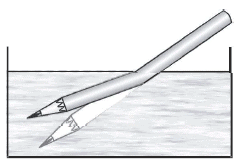Bending of an object: When a point object in a denser medium is seen from a rarer medium it appears to be at a depth d/μ.

Twinkling of stars: Due to fluctuations in refractive index of atmosphere the refraction becomes irregular and the light sometimes reaches the eye and sometimes it does not. This gives rise to twinkling of stars.

Total Internal Reflection (TIR): When light ray travel from denser to rarer medium it bends away from the normal if the angle of incidence is increased angle of refraction will also increased. At a particular value of angle of incidence the refracted ray subtends 900 angle with the normal, this angle of incidence is known as critical angle (θC). If angle of incidence further increases, the ray comes back in the same medium this phenomenon is known as total internal reflection.

Conditions: Angle of incident > critical angle [i > θc]

Light should travel from denser to rarer medium ⇒ Glass to air, water to air, Glass to water.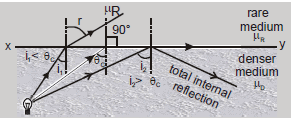Snell's Law at boundary x - y, μsinθC = θR sin 90º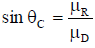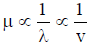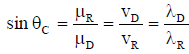When light ray travels from a medium of refractive index m to air then, μR=1, μD = μ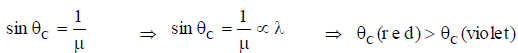For TIR, i > θC ⇒ sin i > sin θC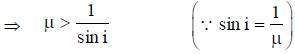When ray travels from glass to air sin θ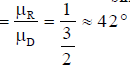⇒ i > 42 degree (TIR), i < 42 degree (refraction)
When ray travel from water to air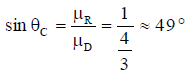i > 49 degree (TIR), i < 49 degree (refraction)
When ray travel from glass to water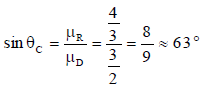i > 63 (TIR) i < 63 (refraction)
A point object is situated at the bottom of a tank filled with a liquid of refractive index m upto height h. It is found that light from the source comes out of liquid surface through a circular portion above the object, then radius and area of circle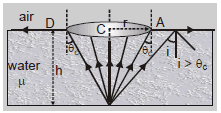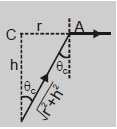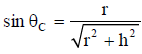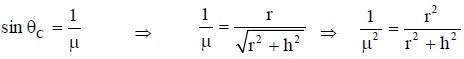μ2r2 = r2 + h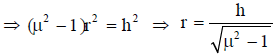area = πr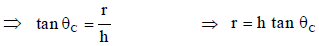Angle which the eye of fish make = 2θC = 2∗49 = 98 . This angle does not depend on depth of liquid.

Some Illustrations of Total Internal Reflection
Sparkling of diamond: The sparkling of diamond is due to total internal reflection inside it. As refractive index for diamond is 2.5, so θC = 24. Now the cutting of diamond is such that i > θC. So TIR will take place again and again inside it. The light which beams out from a few places in some specific directions makes it sparkle.

Optical Fibre: In it, light through multiple total internal reflections is propagated along the axis of a glass fibre of radius of few microns in which index of refraction of core is greater than that of surroundings.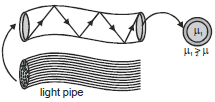Mirage and looming: Mirage is caused by total internal reflection in deserts where due to heating of the earth, refractive index of air near the surface of earth becomes lesser than above it. Light from distant objects reaches the surface of earth with i > θ so that TIR will take place and we see the image of an object along with the object as shown in figure.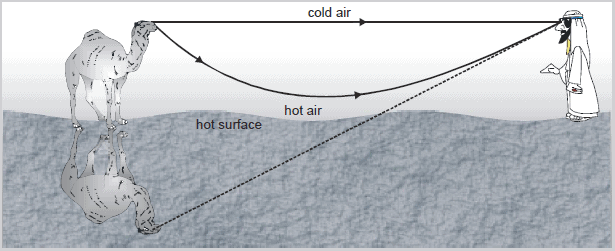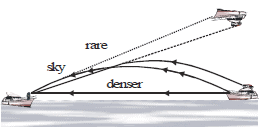Similar to 'mirage' in deserts, in polar regions 'looming' takes place due to TIR. Here m decreases with height and so the image of an object is formed in air if (i>θ) as shown in Fig.

Golden key points

• A diver in water at a depth d sees the world outside through a horizontal circle of radius.
• r = d tanθ.
• For total internal reflection to take place light must be propagating from denser to rarer medium.
• In case of total internal reflection, as all (i.e. 100%) incident light is reflected back into the same medium there is no loss of intensity while in case of reflection from mirror or refraction from lenses there is some of intensity as all light can never be reflected or refracted. This is why images formed by TIR are much brighter than formed by mirrors or lenses.
Offer running on EduRev: Apply code STAYHOME200 to get INR 200 off on our premium plan EduRev Infinity!

,

,

,

,

,

,

,

,

,

,

,

,

,

,

,

,

,

,

,

,

,

;